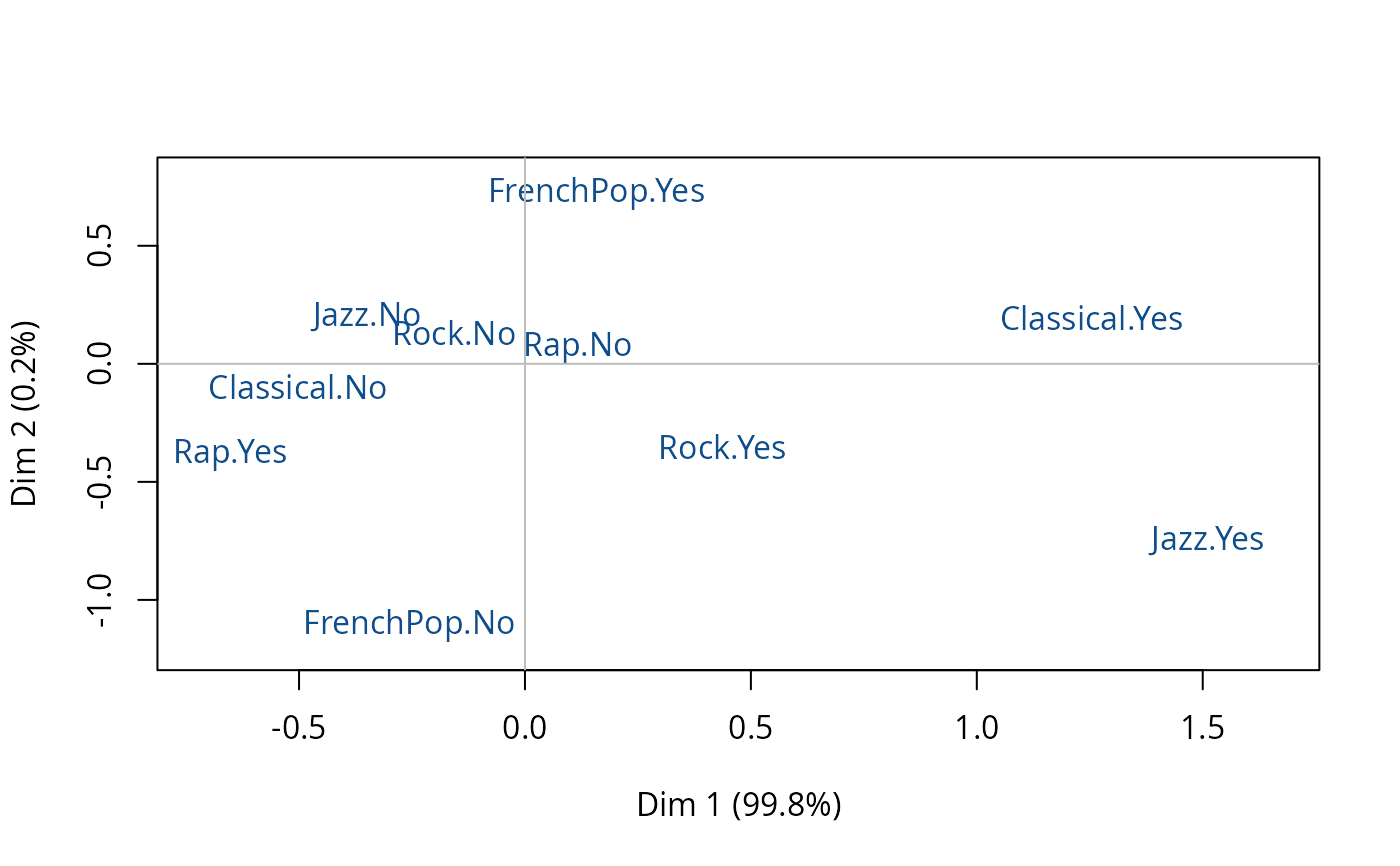Performs a "class specific"" Multiple Correspondence Analysis, i.e. a variant of MCA consisting in analyzing a subcloud of individuals.

csMCA(data, subcloud = rep(TRUE, times = nrow(data)), excl = NULL, ncp = 5,
row.w = rep(1, times = nrow(data)))

## Arguments

data

data frame with n rows (individuals) and p columns (categorical variables)

subcloud

a vector of logical values and length n. The subcloud of individuals analyzed with class specific MCA is made of the individuals with value TRUE.

excl

nnumeric vector indicating the indexes of the "junk" categories (default is NULL). See getindexcat or use ijunk interactive function to identify these indexes. It may also be a character vector of junk categories, specified in the form "namevariable.namecategory" (for instance "gender.male").

ncp

number of dimensions kept in the results (default is 5)

row.w

an optional numeric vector of row weights (by default, a vector of 1 for uniform row weights)

## Details

This variant of MCA is used to study a subset of individuals with reference to the whole set of individuals, i.e. to determine the specific features of the subset. It consists in proceeding to the search of the principal axes of the subcloud associated with the subset of individuals (see references).

## Value

An object of class csMCA, i.e. a list including:

eig

a list of vectors containing all the eigenvalues, the percentage of variance, the cumulative percentage of variance, the modified rates and the cumulative modified rates

call

a list with informations about input data

ind

a list of matrices containing the results for the individuals (coordinates, contributions)

var

a list of matrices containing all the results for the categories and variables (weights, coordinates, squared cosines, categories contributions to axes and cloud, test values (v.test), squared correlation ratio (eta2), variable contributions to axes and cloud

## Author

Nicolas Robette

ijunk, speMCA

## Examples

# class specific MCA of the subcloud of women
# from the Music example data set
data(Music)
junk <- c("FrenchPop.NA", "Rap.NA", "Rock.NA", "Jazz.NA", "Classical.NA")
female <- Music\$Gender=="Women"
mca <- csMCA(Music[,1:5],
subcloud = female,
excl = junk)
plot(mca)